# A Face Detection Method Based on Image Processing and Improved Adaptive Boosting Algorithm

A Face Detection Method Based on Image Processing and Improved Adaptive Boosting Algorithm
School of Information Engineering, Suzhou University, Suzhou 234000, China

Corresponding Author Email:
lixianwei2020@126.com
Page:
395-403
|
DOI:
https://doi.org/10.18280/ts.370306
20 February 2020
|
Accepted:
1 May 2020
|
Published:
30 June 2020
| Citation

OPEN ACCESS

Abstract:

In face detection, the Adaptive Boosting (AdaBoost) algorithm consumes a long training time and faces a high false positive rate. To solve these problems, this paper puts forward an improved AdaBoost face detection method. Firstly, the original image was preprocessed to eliminate the effects of light and noise, improving the image detection effect. Next, a dual threshold weak classifier was designed to replace the single threshold weak classifier. The designed classifier identifies thresholds more accurately and reduce the number of threshold searches, making the algorithm faster in convergence and more efficient in training and detection. Then, the authors optimized the weighting coefficient formula of weak classifiers, focusing on the recognition ability of positive samples and the reliability of weak classifiers. Through the optimization, the algorithm can achieve a low false alarm rate (FAR) under a given low false recognition rate (FRR). After that, two thresholds were used to classify the error range of samples. To increase the weights of large error samples, the original weights of samples were multiplied with different weighting coefficients. In this way, the abnormal samples are more likely to be detected in the next round of training. Simulation results show that the proposed face detection algorithm boasts a high detection accuracy, and consumes a short time in training and detection.

Keywords:

1. Introduction

Face detection, as the basis of face recognition, is a hot topic in computer vision and pattern recognition. It has been widely applied in many fields, ranging from identity (ID) verification, human-machine interface (HCI), visual communication, virtual reality (VR), to public security file management [1-5].

Adaptive Boosting (AdaBoost)  is a popular face detection algorithm, which is excellent in performance and easy to implement. However, AdaBoost algorithm has several defects: it is very time consuming to train the algorithm with a large training set; overfitting may occur during training, undermining the performance of the algorithm [7-11].

To overcome these defects, this paper puts forward an improved AdaBoost face detection method. Firstly, the original image was preprocessed to eliminate the effects of light and noise, improving the image detection effect. To speed up the training of AdaBoost, simple weak classifiers were trained with dual threshold search, instead of the traditional single threshold search. On this basis, strong classifiers were constructed with fewer weak classifiers, which reduces the number of threshold searches, shortens the training time, and increases the detection speed. After that, the weighting coefficients were optimized to solve the problem that the minimal upper limit of error rate does not necessarily lead to the minimal error rate: the weighting coefficient formula was modified to include recognition rate and reliability. Through the modification, the algorithm can achieve a low false alarm rate (FAR) under a given low false recognition rate (FRR). Finally, the original weights of samples were multiplied with different weighting coefficients, making samples with gross error less lightly to be selected to train the weak classifiers in subsequent rounds.

The main contributions of this research are as follows:

(1) The original image was enhanced by histogram equalization, reducing the effects of light on image quality; then, the image quality was further improved through denoising.

(2) The dual threshold method was adopted to reduce the number of weak classifiers in each strong classifier, shorten the training time, and speed up the detection. Simulation results confirm that our algorithm can effectively shorten the training time, simplify classifier structure, and improve detection speed and accuracy.

(3) The weighting coefficients of weak classifiers, which only reflect the error rate, were optimized considering the recognition ability of positive samples and the reliability of weak classifiers. Through the optimization, the AdaBoost strong classifiers cascaded from weak classifiers can achieve a low FAR under a high detection ate.

(4) The error range of samples were delineated by two thresholds. To increase the weights of large error samples, the original weights of samples were multiplied with different weighting coefficients. In this way, the abnormal samples are less likely to be selected in the next round of training.

The remainder of this paper is organized as follows: Section 2 reviews the relevant literature; Section 3 details the image processing; Section 4 improves the AdaBoost algorithm; Section 5 evaluates the effectiveness of our algorithm through simulation; Section 6 puts forward the conclusions.

2. Literature Review

Face detection and recognition are two key tasks in digital image processing [12, 13]. Among them, face detection has been regarded as a key research direction. Most face detection methods are based on either features or statistical rules.

The feature-based methods detect faces in the light of knowledge rules, feature invariance rules or template matching rules. Based on knowledge rules, the face detection methods are not highly efficient . Based on feature invariance rules, the face detection methods cannot capture the facial features well, under the changes of attitude, angle and expression . Based on template matching rules, the face detection methods incur an excessive computing load, making it difficult to establish standard models .

The statistical rule-based methods extract and use the common rules of faces through training on numerous face samples. The most popular statistical rule-based method is AdaBoost . On the upside, Adaboost face detection features high robustness, high detection rate and low FRR. On the downside, the algorithm lacks an optimization mechanism for parameters, and requires lots of samples and long time in training, failing to achieve a good real-time performance.

This paper develops a novel AdaBoost algorithm for face detection. Firstly, the original image was subjected to light treatment and noise removal. Then, the solving formula of weighting coefficients was optimized by adding the weights and parameters that promotes the recognition of positive samples. Through the optimization, the cascade AdaBoost classifiers can achieve a low FAR under a high detection rate. Moreover, the dual threshold method was introduced to reduce the number of weak classifiers that constitute each strong classifier, thereby reducing training time and speeding up the detection. Finally, the original weights of samples were multiplied with different weighting coefficients, making abnormal samples less lightly to be selected in the next round of training. The effectiveness of the improved algorithm was fully demonstrated through simulation.

3. Image Processing

The quality of an image will decrease if the light is too bright or too dark or if the image contains noises. In either case, the effect of face detection will be unsatisfactory . To realize efficient detection, the original image should go through light treatment and noise removal [25-27].

This paper adopts histogram equalization to increase image contrast. In essence, histogram equalization performs nonlinear operation on the inputted image based on the grayscale mapping relationship. Through the operation, the equalized image pixels are distributed evenly across multiple grayscales. Compared with the original image, the equalized image has a clear contrast and a large dynamic range of grayscales.

Figure 1 provides an original image with a bright background and its equalized image. Figure 2 provides an original image with a dark background and its equalized image. From the two figures, it can be seen that histogram equalization clearly improved the quality of the two images.

The acquisition of face images is inevitably disturbed by noises. The noise interference reduces image quality, making it difficult to recognize facial features. To improve facial detection, it is necessary to denoise the original image.

There are three common types of image noises, namely, Gaussian noise, salt-and-pepper noise and Poisson noise. In this paper, Gaussian noise in an original image is removed with Gaussian filter (Figure 3), salt-and-pepper noise with median filter (Figure 4), and Poisson noise with mean filter (Figure 5).

## 1.1.pngFigure 1. (a) Original image with a bright background and (b) its equalized image

## 1.2.pngFigure 2. (a) Original image with a dark background and (b) its equalized image

## 1.3.pngFigure 3. (a) Original image; (b) Image with Gaussian noise; (c) Image processed by Gaussian filter

## 1.4.pngFigure 4. (a) Original image; (b) Image with salt-and-pepper noise; (c) Image processed by median filter

As shown in Figures 3-5, the three kinds of image noises were effectively removed by the corresponding filter. Taking a pixel as the starting point, the Gaussian filter computes the weight of another pixel based on the Euclidean distance from the pixel to the starting point. The median filter is good at removing salt-and-pepper noise, for the pixels distorted by this noise tend to have an excessively large or small grayscale. The mean filter is a desirable tool to eliminate the Poisson noise.

## 1.5.pngFigure 5. (a) Original image; (b) Image with Poisson noise; (c) Image processed by mean filter

4. Face Detection Based on Improved AdaBoost Algorithm

In AdaBoost algorithm, weak classifiers are developed through sample training. Then, the most powerful weak classifiers are selected based on Haar-like eigenvalues, and cascaded into strong classifiers [28, 29]. By classification ability, the strong classifiers are ranked in ascending order (Figure 6).

In actual target detection problems, only a small portion of samples are positive. Under the cascade structure, most samples are filtered out by the classifiers at the front end. Only a few samples can pass through all the classifiers on different stages. Therefore, the cascade structure greatly simplifies the computation, and promotes the efficiency of face detection.

## 1.6.png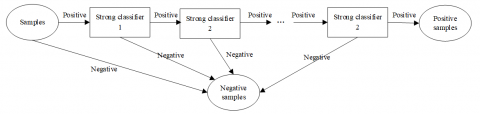The workflow of AdaBoost algorithm can be described as follows:

Step 1. Input the training set: (x1, y1), (x2, y2), ..., (xn, yn), where $x_{i} \in X, y_{i} \in Y=\{-1,1\}$.

Step 2. Initialize sample weights: w1(i)=1/n;

Step 3. Iterate t=1, 2, ..., T times and search for T weak classifiers ht;

(a) For the j-th Haar-like feature of each sample, design a simple classifier hj:

${{h}_{j}}(x)=\left\{ \begin{matrix} 1 & {{p}_{j}}{{f}_{j}}(x)<{{p}_{j}}{{\delta }_{j}} \\ 0 & \text{ otherwise } \\\end{matrix} \right.$     (1)

where, fj(x) is the j-th eigenvalue; δj is the treshold; pj is the bias that determines the sign of the inequality (pj=+1 or -1). Then, adjsut δj and pj to minimize the weighted error rate εj:

${{\varepsilon }_{j}}=\sum\limits_{{{h}_{j}}\left( {{x}_{i}} \right)\ne {{y}_{i}}}{{{\text{w}}_{j}}}(i)$      (2)

(b) Select the smallest εt from all εj values to serve as the weak classifier ht of the current iteration.

(c) Solve the weighting coefficient of ht:

${{\alpha }_{t}}=\frac{1}{2}\ln \left( \frac{1-{{\varepsilon }_{t}}}{{{\varepsilon }_{t}}} \right)$    (3)

(d) Update sample weights:

${{w}_{t+1}}(i)=\frac{{{w}_{t}}(i)\exp \left( -{{\alpha }_{t}}{{y}_{i}}{{h}_{t}}\left( {{x}_{i}} \right) \right)}{{{z}_{t}}}$     (4)

where, Zt is the normalization factor:

${{Z}_{t}}=\sum\limits_{i}{{{w}_{t}}}(i)\exp \left( -{{\alpha }_{t}}{{y}_{i}}{{h}_{t}}\left( {{x}_{i}} \right) \right)$       (5)

Step 4. Combine weak classifiers into a strong cascade classifier:

$H(x)=\left\{ \begin{matrix} 1 & \sum\limits_{t=1}^{T}{{{\alpha }_{t}}}(i){{h}_{t}}(x)\ge 0.5\sum\limits_{t=1}^{T}{{{\alpha }_{t}}} \\ 0 & \text{ otherwise } \\\end{matrix} \right.$      (6)

where, $\alpha_{t}=\log \left(\frac{1}{\beta_{t}}\right)$.

4.2.1 Expanded sample library

The detection effect of AdaBoost algorithm hinges on the diversity of training samples [30, 31]. The algorithm will perform poorly in detection, if the type of samples to be detected is not contained in the training set. The larger and more diverse the sample library, the better the training result.

To diversify training samples, this paper adds the translation, rotation and mirror images (Figure 7) of each sample to the sample library. The detection result will be improved, if the expanded library contains the type of samples to be detected. Therefore, the types of training samples were selected depending on those of the images to be detected.

## 1.7.pngFigure 7. (a) Original image; (b) Translation image; (c) Rotation image; (d) Mirror image

## 1.8.png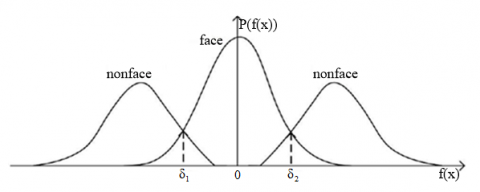Figure 8. Distribution of face and nonface samples

4.2.2 Improved construction method for weak classifiers

(1) Determining weak classifiers by dual threshold method

In Step 3 of AdaBoost algorithm, a simple classifier hj (j=1, 2, ..., l) for l features should be solved to build a weak classifier ht. Each simple classifier hj needs to search M times, where M is the number of samples. Then, T weak classifiers are needed to form a strong classifier. Suppose l=20,000 and T= 200, and it takes 0.5s to train a simple classifier hj. Then, the total training lasts 20,000×0.5×200≈555.5h, that is, 23 days.

The accuracy of AdaBoost algorithm is positively correlated with the size and diversity of the training library. Hence, a long training time is necessary to improve the detection accuracy. To shorten the excessively long training time, the original AdaBoost algorithm was improved as follows.

Figure 8 above shows the eigenvalue distribution of face and nonface samples. It can be seen that face and nonface images are distributed unevenly. The face images are at the center, while nonface images are on two sides. In the AdaBoost algorithm, each weak classifier only has a threshold as the upper or lower limit of the eigenvalue. Under the above distribution, dual threshold simple classifier has better classification effect.

Therefore, the dual threshold method was adopted to solve weak classifiers, that is, two thresholds were taken as the upper and lower limits of each weak classifier. Each dual threshold weak classifier is equivalent to two single-threshold weak classifiers. In this way, the number of weak classifiers is reduced, and the training time is shortened.

The specific process is detailed below:

Step 1. Determine the distribution curves of face and nonface images f1(x) and f2(x), where is the eigenvalue.

Step 2. Solve the xmax corresponding to the maximum value of f1(x)-f2(x).

Step 3. Search for x1 and x2 that make f1(x)=f2(x) from xmax to the left and right. If the two values are found, the two thresholds of the weak classifier: δ1=x1 and δ2=x2; otherwise, take the boundary points as the thresholds.

(2) Optimzing the weighting coefficient formula

In traditional AdaBoost algorithm, the weighting coefficients of weak classifiers are solved based on the upper limit of minimum error rate. If the FRR is low, the algorithm cannot achieve a good performance. Therefore, the weighting coefficient formula is optimized in this research.

The weighting coefficients of weak classifiers should not be optimized solely based on error rate. Since AdaBoost mainly recognizes the classes of samples, the recognition ability of positive samples and the reliability of weak classifiers should also be considered to combine weak classifiers into strong classifiers. The reliability equals the recognition rate divided by acceptance rate. Taking these two factors into account, the weighting coefficients can be solved by:

${{\alpha }_{t}}=\frac{1}{2}\ln \left( \frac{1-{{\varepsilon }_{t}}}{{{\varepsilon }_{t}}} \right)+k\cdot \frac{\left( 1-{{\varepsilon }_{t}} \right)}{\rho }\cdot \left( 1-{{\varepsilon }_{t}} \right)$     (7)

where, k is a constant that lowers the upper limit of the minimum error rate in the t-th iteration (multiple tests have shown that k=1/120 brings the best detection effect); ρ is the acceptance rate of positive samples ($\rho=\frac{N_{c p}}{N_{p}+N_{n}}$, where Ncp is the number of correctly classified positive samples; Np and Nn are the number of positive and negative samples in the sample set, respectively). When the error rate remains the same, the weak classifiers with stronger recognition ability of positive samples and higher reliability should be assigned a higher weight.

The following proves that the optimized weighting coefficient formula can improve the H(xi) value distirbution of samples, and thus promote the performance of the weak classifiers.

Assuming that $\gamma=\frac{\left(1-\varepsilon_{t}\right)^{2}}{\rho}$, the weighted sum of H(xi) corresponding to sample xi can be obtained as:

\begin{align} & H\left( {{x}_{\text{i}}} \right)=\sum\limits_{t}{{{\alpha }_{t}}}{{h}_{t}}\left( {{x}_{i}} \right)=\sum\limits_{t}{\left[ \frac{1}{2}\ln \left( \frac{1-{{\varepsilon }_{t}}}{{{\varepsilon }_{t}}} \right)+\gamma \right]}{{h}_{t}}\left( {{x}_{i}} \right) \\ & =\sum\limits_{t}{\frac{1}{2}}{{h}_{t}}\left( {{x}_{i}} \right)\ln \left( \frac{1-{{\varepsilon }_{t}}}{{{\varepsilon }_{t}}} \right)+\sum\limits_{t}{\gamma }{{h}_{t}}\left( {{x}_{i}} \right) \\\end{align}     (8)

The first term on the right side of formula (8) is the same as the traditional AdaBoost algorithm. Thus, the following focuses on the second term on that side. Assuming that $H_{\Delta}\left(x_{\mathrm{i}}\right)=\sum_{t} \gamma h_{t}\left(x_{i}\right)$, the positive samples satisfy:

$\sum\limits_{{{y}_{i}}=1}{{{H}_{\Delta }}}\left( {{x}_{i}} \right)=\sum\limits_{{{y}_{i}}=1}{\gamma }\sum\limits_{t}{{{h}_{t}}}\left( {{x}_{i}} \right)$     (9)

The corresponding negative samples satisfy:

$\sum\limits_{{{y}_{i}}=-1}{{{H}_{\Delta }}}\left( {{x}_{i}} \right)=\sum\limits_{{{y}_{i}}=-1}{\gamma }\sum\limits_{t}{{{h}_{t}}}\left( {{x}_{i}} \right)$     (10)

Let (Np+Nn)ρ and Np-(Np+Nn)ρ be the number of positive samples correctly and incorrectly recognized in the t-th iteration, respectively. Then, we have:

$\sum_{y_{i}=1} h_{t}\left(x_{i}\right)$$=\left(N_{p}+N_{n}\right) \rho \cdot 1+\left[N_{p}-\left(N_{p}+N_{n}\right) \rho\right] \cdot(-1)$$=2\left(N_{p}+N_{n}\right) \rho-N_{p}$     (11)

Substituting formula (11) into formula (9), we have:

\begin{align} & \sum\limits_{{{y}_{i}}=1}{{{H}_{\Delta }}}\left( {{x}_{i}} \right)=\sum\limits_{t}{\gamma }\sum\limits_{{{y}_{i}}=1}{{{h}_{t}}}\left( {{x}_{i}} \right) \\ & =\sum\limits_{t}{\gamma }\left[ 2\left( {{N}_{p}}+{{N}_{n}} \right)\rho -{{N}_{p}} \right] \\\end{align}    (12)

Let $\left(N_{p}+N_{n}\right) \cdot \varepsilon_{t}$ be the total number of samples incorrectly recognized by the t-th weak classifier. Then, the number of incorrectly recognized samples can be expressed as $\left(N_{p}+N_{n}\right) \cdot \varepsilon_{t}-\left[N_{p}-\left(N_{p}+N_{n}\right) \rho\right] .$ Hence, the number of incorrectly recognized negative samples can be obtained as: $N_{n}-\left\{\left(N_{p}+N_{n}\right) \cdot \varepsilon_{t}-\left[N_{p}-\left(N_{p}+N_{n}\right) \rho\right]\right\} .$ Then, we have:

\begin{align} & \sum\limits_{{{y}_{i}}=-1}{{{h}_{t}}}\left( {{x}_{i}} \right) \\ & =\left\{ {{N}_{n}}-\left[ \left( {{N}_{p}}+{{N}_{n}} \right)\cdot {{\varepsilon }_{t}}-\left[ {{N}_{p}}-\left( {{N}_{p}}+{{N}_{n}} \right)\rho \right] \right] \right\}\cdot (-1) \\ & +\left\{ \left( {{N}_{p}}+{{N}_{n}} \right)\cdot {{\varepsilon }_{t}}-\left[ {{N}_{p}}-\left( {{N}_{p}} \right) \right. \right.\left. \left. \left. +{{N}_{n}} \right)\rho \right] \right\}\cdot 1 \\ & =2\left( {{N}_{p}}+{{N}_{n}} \right)\cdot {{\varepsilon }_{t}}+2\left( {{N}_{p}}+{{N}_{n}} \right)\rho -\left( 2{{N}_{p}}+{{N}_{n}} \right) \\\end{align}      (13)

If εt<0.5 during the training, the following can be derived from formula (13):

\begin{align} & \sum\limits_{{{y}_{i}}=-1}{{{h}_{t}}}\left( {{x}_{i}} \right) \\ & <2\cdot \left( {{N}_{p}}+{{N}_{n}} \right)\cdot 0.5+2\left( {{N}_{p}}+{{N}_{n}} \right)\rho -\left( 2{{N}_{p}} \right.\left. +{{N}_{n}} \right) \\ & ={{N}_{p}}+{{N}_{n}}-2{{N}_{p}}-{{N}_{n}}+2\left( {{N}_{p}}+{{N}_{n}} \right)\rho \\ & =2\left( {{N}_{p}}+{{N}_{n}} \right)\rho -{{N}_{p}} \\\end{align}       (14)

From formulas (10), (12), and (14), we have:

\begin{align} & \sum\limits_{{{y}_{i}}=1}{{{H}_{\Delta }}}\left( {{x}_{i}} \right)=\sum\limits_{t}{\gamma }\sum\limits_{{{y}_{i}}=-1}{{{h}_{t}}}\left( {{x}_{i}} \right) \\ & <\sum\limits_{t}{\gamma }\left[ 2\left( {{N}_{p}}+{{N}_{n}} \right)\rho -{{N}_{p}} \right]=\sum\limits_{{{y}_{i}}=1}{{{H}_{\Delta }}}\left( {{x}_{i}} \right) \\\end{align}       (15)

The above theoretical analysis proves that the optimized weighting coefficient formula meets the detection requirements. By extending the distribution of positive and negative samples to both sides, the trained classifiers can realize a lower FAR, when the FRR is fixed. Under a constant error rate, the classifiers with a stronger ability to recognize positive samples are given greater weighting coefficeints, further improving the face detection rate of the classifiers.

(3) Updating sample weights with two thresholds

The sample weight update formula (4) was improved as follows:

${{w}_{t+1}}(i)=\left\{ \begin{matrix} \frac{{{w}_{t}}(i)\exp \left( -{{\alpha }_{t}}{{y}_{i}}{{h}_{t}}\left( {{x}_{i}} \right) \right)}{{{Z}_{t}}},{{e}_{t}}(i)\le {{\varepsilon }_{t}} \\ {{\beta }_{1}}\times \frac{{{w}_{t}}(i)\exp \left( -{{\alpha }_{t}}{{y}_{i}}{{h}_{t}}\left( {{x}_{i}} \right) \right)}{{{z}_{t}}},{{\varepsilon }_{t}}<{{e}_{t}}(i)\le 2{{\varepsilon }_{t}} \\ {{\beta }_{2}}\times \frac{{{w}_{t}}(i)\exp \left( -{{\alpha }_{t}}{{y}_{i}}{{h}_{t}}\left( {{x}_{i}} \right) \right)}{{{z}_{t}}},{{e}_{t}}(i)>2{{\varepsilon }_{t}} \\\end{matrix} \right.$      (16)

where, β1 and β2 are two weight adjustment factors; et(i) is the relative error of a weak classifier:

${{e}_{t}}(i)=\left| \frac{{{h}_{t}}\left( {{x}_{i}} \right)-{{y}_{i}}}{{{y}_{i}}} \right|,i=1,2,3,\cdots n$      (17)

If the relative error et(i) of training samples is more than twice the weighted error rate, the samples must contain a gross error. These samples should be given a smaller weight, making them less likely to be selected to train weak classifiers in the next round. Hence, the weights of such samples should be multiplied by a smaller-than-one adjustment factor β2=0.78.

If the relative error et(i) of training samples is less than twice the weighted error rate, the samples must contain a large stochastic error. These samples should be given a larger weight, making them more likely to be selected to train weak classifiers in the next round. Hence, the weights of such samples should be multiplied by a greater-than-one adjustment factor β1=1.1.

The weight update limits the weight divergence of weak classifiers, thereby improving the accuracy of face detection.

(4) Implementing the improved AdaBoost algorithm

The improvement mainly focuses on Step 3 on the traditional algorithm. Steps 1, 2, and 3 are the same as the traditional steps. The improved version of Step 3 is detailed below:

Step 3. Iterate t=1, 2, ..., T times and search for T weak classifiers ht;

(a) For the j-th Haar-like feature of each sample, design a simple classifier hj through training by dual threshold method, that is, find the thresholds δ1 and δ2 that minimize weighted error rate \varepsilon_{j}=\sum_{h_{j}\left(x_{i}\right) \neq y_{i}} \mathrm{w}_{t}(j), and compute the relative absolute error of weak classifiers ej(i):

${{h}_{j}}(x)=\left\{ \begin{matrix} 1 & {{\delta }_{1}}<{{f}_{j}}(x)<{{\delta }_{2}} \\ 0 & \text{ otherwise } \\ \end{matrix} \right.$    (18)

(b) Select the smallest εt from all εj values to serve as the weak classifier ht of the current iteration.

(c) Solve the weighting coefficient of ht by formula (7).

(d) Update sample weights by formula (16).

5. Simulation

To verify its effectiveness, the improved AdaBoost algorithm was simulated on MIT and Yale’s face and nonface image databases. The face database contains 2,429 face samples (pixel size: 19×19), and the nonface database contains 4,548 nonface samples (pixel size: 19×19). The simulation was carried out on MATLAB 2017, which runs on a computer with a 3GHz CPU and 8GB memory.

Figure 9 compares the convergence effects of the traditional and improved AdaBoost algorithms on the training set. It can be seen that the improved algorithm converged much faster than the traditional algorithm on the training set. The main reason is that the improved algorithm replaces the single threshold with two thresholds, and simplifies the cascade structure of AdaBoost strong classifiers.

Figure 10 compares the receiver operating characteristic (ROC) curves of the traditional and improved AdaBoost algorithms on the test set. To reflect the FAR decline of our algorithm at low-FRR end, the ROC in Figure 10 was intercepted from the curve in the low-FRR section. Obviously, on the high detection end (low-FRR end), the improved algorithm achieved better FAR, thanks to the optimization of the weighting coefficient formula, which considers the recognition ability of positive samples and the reliability of weak classifiers.

The face detection performance of both algorithms was evaluated by Precision and Recall. The greater the Precision, the more accurate the detection; the higher the Recall, the more complete the detection. Figures 11 and 12 compare the Precisions and Recalls of the two algorithms. The comparison shows that the improved algorithm had much higher Precision and Recall than the traditional algorithm.

## 1.9.jpg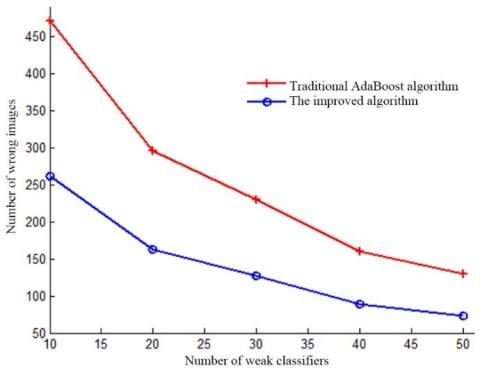Figure 9. Comparison of convergence effect

## 10.png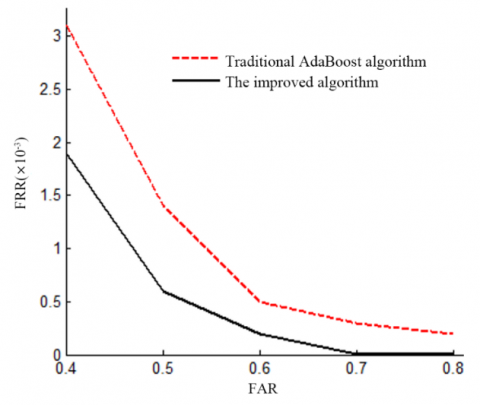Figure 10. Comparison of ROC curves

## 11.png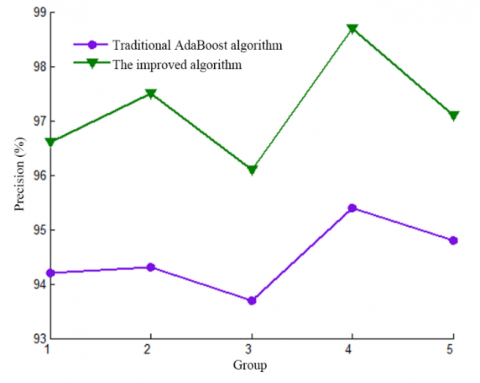Figure 11. Comparison of precisions

## 12.png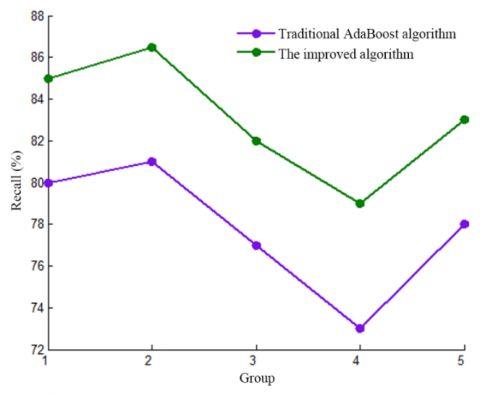Figure 12. Comparison of recalls

## 13.pngFigure 13. Comparison of detection results

## 14.pngFigure 14. Comparison of running time

Next, 56 weak classifiers were combined into strong classifiers by each algorithm, and used to detect faces in the same image. The detect results (Figure 13) show that the improved algorithm achieved more accurate detection than the traditional algorithm. The traditional algorithm mistook many nonfaces for faces (Figure 13a). These mistakes were corrected by the improved algorithm, leading to a low FRR. Of course, both algorithms failed to detect some faces, due to the small number of classifiers.

Finally, the running time of both algorithms are compared in Figure 14. It is clear that the improved algorithm is more efficient than the traditional algorithm, and the advantage increase with the number of training samples.

6. Conclusions

This paper designs and verifies an improved AdaBoost algorithm for face detection. Firstly, the original image quality was improved through light treatment and noise removal, and the training set was expanded to enhance detection accuracy. Next, the dual threshold method was introduced to reduce the number of searches, which indirectly shortens the training time and improves the detection speed. After that, the weighting coefficient formula was optimized, ensuring that the classifiers can achieve a low FAR under a high detection rate. Finally, the sample weights were updated with two thresholds. The improved algorithm requires a much shorter training time than the traditional AdaBoost algorithm, especially when there are lots of training samples. Simulation results show that our algorithm greatly improves the training speed and detection effect of the traditional algorithm. The future research will extend the algorithm to multi-class object detection, and further improve the training speed and detection accuracy of the algorithm.

Acknowledgment

This work was supported by outstanding academic and technical backbone of Suzhou University (Grant No.: 2016XJGG12), the third batch of reserve candidates for academic and technical leaders (Grant No.: 2018XJHB07), Suzhou science and technology project (Grant No.: SZ2018GG01, SZ2018GG01xp), Collaborative education project (Grant No.: 201902167037, 201902167019), Key curriculum construction project (Grant No.: szxy2018zdkc19), Large scale online open course (MOOC) demonstration project (Grant No.: 2019mooc300, 2019mooc318), Professional leader of Suzhou University (Grant No.: 2019XJZY22), Anhui province's key R&D projects include Dabie Mountain and other old revolutionary base areas, Northern Anhui and poverty-stricken counties in 2019 (Grant No.: 201904f06020051).

References

 Gundogdu, B., Bianco, M.J. (2020). Collaborative similarity metric learning for face recognition in the wild. IET Image Processing, 14(9): 1759-1768. https://doi.org/10.1049/iet-ipr.2019.0510

 Du, L., Hu, H., Wu, Y. (2019). Cycle age-adversarial model based on identity preserving network and transfer learning for cross-age face recognition. IEEE Transactions on Information Forensics and Security, 15: 2241-2252. https://doi.org/10.1109/TIFS.2019.2960585

 Fekri-Ershad, S. (2019). Gender classification in human face images for smart phone applications based on local texture information and evaluated Kullback-Leibler divergence. Traitement du Signal, 36(6): 507-514. https://doi.org/10.18280/ts.360605

 Yu, B., Tao, D. (2018). Anchor cascade for efficient face detection. IEEE Transactions on Image Processing, 28(5): 2490-2501. https://doi.org/10.1109/TIP.2018.2886790

 Pei, J.Y., Shan, P. (2019). A micro-expression recognition algorithm for students in classroom learning based on convolutional neural network. Traitement du Signal, 36(6): 557-563. https://doi.org/10.18280/ts.360611

 Freund, Y., Schapire, R.E. (1995). A desicion-theoretic generalization of on-line learning and an application to boosting. In European Conference on Computational Learning Theory, pp. 23-37. https://doi.org/10.1007/3-540-59119-2_166

 Tsai, Y.H., Lee, Y.C., Ding, J.J., Chang, R.Y., Hsu, M.C. (2018). Robust in-plane and out-of-plane face detection algorithm using frontal face detector and symmetry extension. Image and Vision Computing, 78: 26-41. https://doi.org/10.1016/j.imavis.2018.07.003

 Subasi, A., Kremic, E. (2020). Comparison of AdaBoost with multiboosting for phishing website detection. Procedia Computer Science, 168: 272-278. https://doi.org/10.1016/j.procs.2020.02.251

 Chen, Y., Chen, W.G., Wang, X., Yu, R., Tian, Y. (2019). Learning-based method for lane detection using regionlet representation. IET Intelligent Transport Systems, 13(12): 1745-1753. https://doi.org/10.1049/iet-its.2019.0015

 Chen, G., Hong, Z., Guo, Y., Pang, C. (2019). A cascaded classifier for multi-lead ECG based on feature fusion. Computer Methods and Programs in Biomedicine, 178: 135-143. https://doi.org/10.1016/j.cmpb.2019.06.021

 Qu, H., Feng, T., Wang, Y., Zhang, Y. (2019). AdaBoost-SCN algorithm for optical fiber vibration signal recognition. Applied Optics, 58(21): 5612-5623. https://doi.org/10.1364/AO.58.005612

 Liu, C., Chang, F. (2018). Hybrid cascade structure for license plate detection in large visual surveillance scenes. IEEE Transactions on Intelligent Transportation Systems, 20(6): 2122-2135. https://doi.org/10.1109/TITS.2018.2859348

 Qu, H., Feng, T., Wang, Y., Zhang, Y. (2019). AdaBoost-SCN algorithm for optical fiber vibration signal recognition. Applied Optics, 58(21): 5612-5623. https://doi.org/10.1364/AO.58.005612

 Lee, Y. B., Lee, S. (2011). Robust face detection based on knowledge-directed specification of bottom-up saliency. Etri Journal, 33(4): 600-610. https://doi.org/10.4218/etrij.11.1510.0123

 George, M., Sivan, A., Jose, B.R., Mathew, J. (2019). Real-time single-view face detection and face recognition based on aggregate channel feature. International Journal of Biometrics, 11(3): 207-221. https://doi.org/10.1504/IJBM.2019.100829

 Li, H., Hua, G., Lin, Z., Brandt, J., Yang, J. (2013). Probabilistic elastic part model for unsupervised face detector adaptation. In Proceedings of the IEEE International Conference on Computer Vision, pp. 793-800. https://doi.org/10.1109/ICCV.2013.103

 Lee, W., Jun, C.H., Lee, J.S. (2017). Instance categorization by support vector machines to adjust weights in AdaBoost for imbalanced data classification. Information Sciences, 381: 92-103. https://doi.org/10.1016/j.ins.2016.11.014

 Li, C., Ding, X.Q., Wu, Y.T. (2007). A revised AdaBoost algorithm——AD AdaBoost. Chinese Journal of Computers, (01): 105-111. https://doi.org/10.3321/j.issn:0254-4164.2007.01.012

 Dou, P., Chen, Y. (2017). Remote sensing imagery classification using AdaBoost with a weight vector (WV AdaBoost). Remote Sensing Letters, 8(8): 733-742. https://doi.org/10.1080/2150704X.2017.1319987

 Zhang, C.J., Fan, W. (2011). AdaBoost face detection algorithm based on correlation. Computer Engineering, 37(8): 158-163. https://doi.org/10.3969/j.issn.1000-3428.2011.08.054

 Zhang, M.Y., Wang, D.F., Wei, Z.S. (2017). An improved AdaBoost training algorithm. Journal of Northwestern Polytechnical University, 35(6): 1119-1124. https://doi.org/10.3969/j.issn.1000-2758.2017.06.028

 Taherkhani, A., Cosma, G., McGinnity, T.M. (2020). AdaBoost-CNN: An adaptive boosting algorithm for convolutional neural networks to classify multi-class imbalanced datasets using transfer learning. Neurocomputing, 404(3): 351-366. https://doi.org/10.1016/j.neucom.2020.03.064

 Wang, L., Lv, S.X., Zeng, Y.R. (2018). Effective sparse AdaBoost method with ESN and FOA for industrial electricity consumption forecasting in China. Energy, 155: 1013-1031. https://doi.org/10.1016/j.energy.2018.04.175

 Windeatt, T., Zor, C., Camgoz, N.C. (2018). Approximation of Ensemble Boundary Using Spectral Coefficients. IEEE Transactions on Neural Networks and Learning Systems, 30(4): 1272-1277. https://doi.org/10.1109/TNNLS.2018.2861579

 Guan, W., Wu, Y., Xie, C., Fang, L., Liu, X., Chen, Y. (2018). Performance analysis and enhancement for visible light communication using CMOS sensors. Optics Communications, 410: 531-551. https://doi.org/10.1016/j.optcom.2017.10.038

 Singh, V., Dev, R., Dhar, N.K., Agrawal, P., Verma, N.K. (2018). Adaptive type-2 fuzzy approach for filtering salt and pepper noise in grayscale images. IEEE Transactions on Fuzzy Systems, 26(5): 3170-3176. https://doi.org/10.1109/TFUZZ.2018.2805289

 Mukam, J.D., Tambue, A. (2019). Optimal strong convergence rates of numerical methods for semilinear parabolic SPDE driven by Gaussian noise and Poisson random measure. Computers & Mathematics with Applications, 77(10): 2786-2803. https://doi.org/10.1016/j.camwa.2019.01.01

 Wang, S.H., Li, H.F., Zhang, Y.J., Zou, Z.S. (2019). A hybrid ensemble model based on ELM and improved AdaBoost. RT algorithm for predicting the iron ore sintering characters. Computational Intelligence and Neuroscience, 1-11. https://doi.org/10.1155/2019/4164296

 Chen, X., Liu, L., Deng, Y., Kong, X. (2019). Vehicle detection based on visual attention mechanism and AdaBoost cascade classifier in intelligent transportation systems. Optical and Quantum Electronics, 51(8): 263. https://doi.org/10.1007/s11082-019-1977-7

 Lin, S., Yu, Q., Wang, Z., Feng, D., Gao, S. (2019). A fault prediction method for catenary of high-speed rails based on meteorological conditions. Journal of Modern Transportation, 27(3): 211-221. https://doi.org/10.1007/s40534-019-0191-4

 Wang, F., Li, Z., He, F., Wang, R., Yu, W., Nie, F. (2019). Feature learning viewpoint of AdaBoost and a new algorithm. IEEE Access, 7: 149890-149899. https://doi.org/10.1109/ACCESS.2019.2947359